Home » FAQ » Is input current of VFD smaller than output current?

# Is input current of VFD smaller than output current?

Input current of the VFD is related to the power required by the motor, the supply voltage, the efficiency of variable frequency drive and the power factor. Among, the power factor of VFD changes along with the impedance of power supply, low impedance leads to low power factor and high impedance leads to high power factor. As the load of VFD is a motor, the input and output power factors are different, the power factor at output end after using the VFD is higher than that of the input end.

Current of the motor depends on the required shaft power, and power factor of motor is usually lower than that of VFD. Due to this feature, the input current of VFD will be smaller than that of the motor driven by it. For example, a 15 kW 380V 4-pole motor, which is driven by a 15 kW (20 hp) three-phase VFD, related parameters are calculated as follows: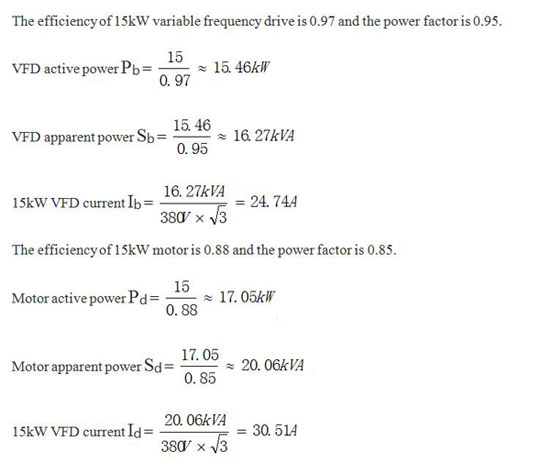Obviously, it is objective that the input current of VFD is smaller than the output current, and someone think the following factors would lead to such a result.

Output current of the variable frequency drive is provided by the inverter. The waveform of current through pulse-width modulation is approximately sinusoidal, and the load requirements can be fulfilled only by increasing output, which is generally larger than 10% of input.

When the VFD is running, the input and output currents contain higher harmonics, it’s difficult to measure the phase angle and errors occur in traditional measurement methods. Errors actually exist in measuring current and power consumption with harmonic components by conventional instruments. However, regardless of the measurement results, the input power factor of VFD is generally higher, which is above 0.95, input power factor of motor is generally about 0.85. According to the conservation of energy, only when the output current is larger than the input current can it satisfy the balance of equation.

Plz Calculate (3 - 4) =
(Your comment will show after approved.)

You may also like:Variable Frequency Drives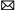info@variablefrequencydrive.net

Featured Articles
Variable Frequency Drive for Fans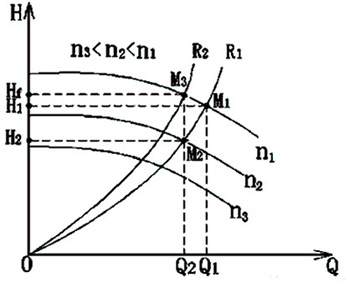Fan is a kind of machine that compresses and conveys gas. If the pressure of exhausted air is quite low, the fan plays a role of ...
Variable Frequency Drive for Food ...Nowadays the food machinery industry has been rapidly developed. As the motor drive, variable frequency drive will change the ...
Can I Run a 60Hz Motor on 50Hz by ...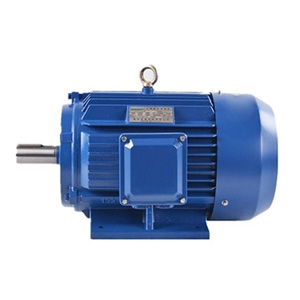We are often asked “can the motor with a rated frequency of 60Hz operate at 50Hz (vice versa)?” When the motor is operated at a ...
How Does Variable Frequency Drive ... The rotating speed of inductor type AC motor (hereinafter referred to as motor) is determined by the number of pole and ...
What is a Variable Frequency Drive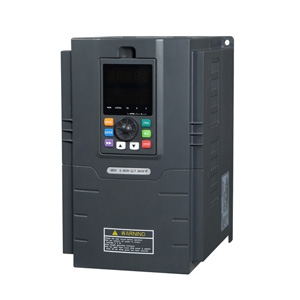Variable frequency drive (VFD) is an adjustable speed drive used for motor drive system, control the speed and torque of the AC ...